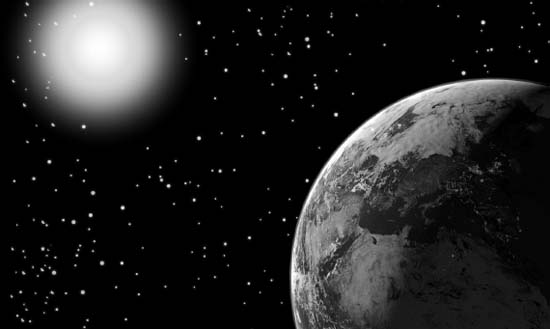NextPrevious

# Why do mathematicians and scientists often use scientific notation?

Scientific notation is a way of making larger and smaller numbers used in the scientific field easier to write, read, and take up less space in calculations. Scientists generally pick the power of ten that is multiplied by a number between 1 and 10 to express these numbers. For example, it is easier to write 0.00023334522 as 2.3334522 × 10–4. To apply this to a scientific example, take the case of the average distance between the Earth and Sun, written in scientific notation as 9.3 × 107 miles—mathematically much easier to work with than the larger number it represents.

The following are several examples of large and small numbers in powers of ten notation similar to numbers used in scientific notation (note: numbers that are extremely small in scientific notation will have negative exponents):

748,000 can be represented by 7.48 × 105

245 can be represented by 2.45 × 102

-45,000 can be represented by -4.5 × 104

0.025 can be represented by 2.5 × 10-2

-0.0036 can be represented by -3.6 × 10-3

0.0000409 can be represented by 4.09 × 10-5

0.0000000014 can be represented by 1.4 × 10-9The average distance between the Earth and the Sun is most easily expressed using powers of ten as 9.3 × 107.

Close

This is a web preview of the "The Handy Math Answer Book" app. Many features only work on your mobile device. If you like what you see, we hope you will consider buying. Get the App